LearningNumber Theory (MOD) Popular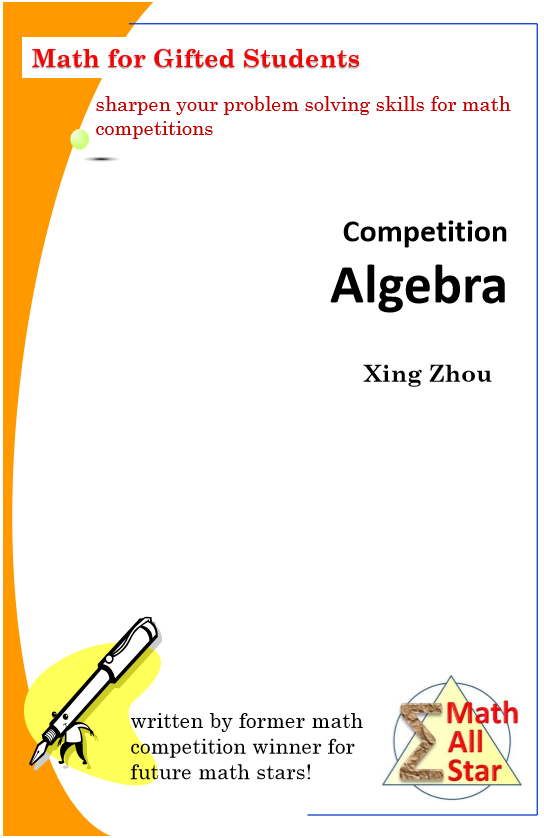Competition Algebra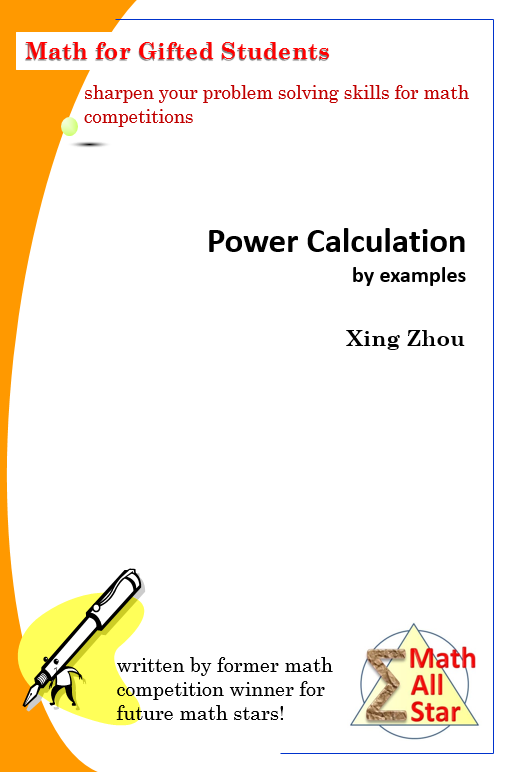Power Calculation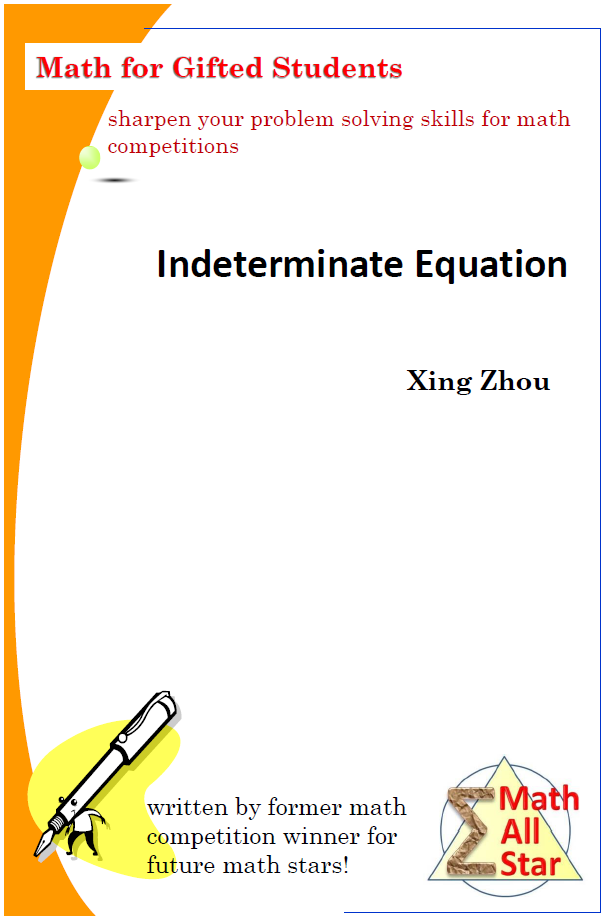Indeterminate Equation popular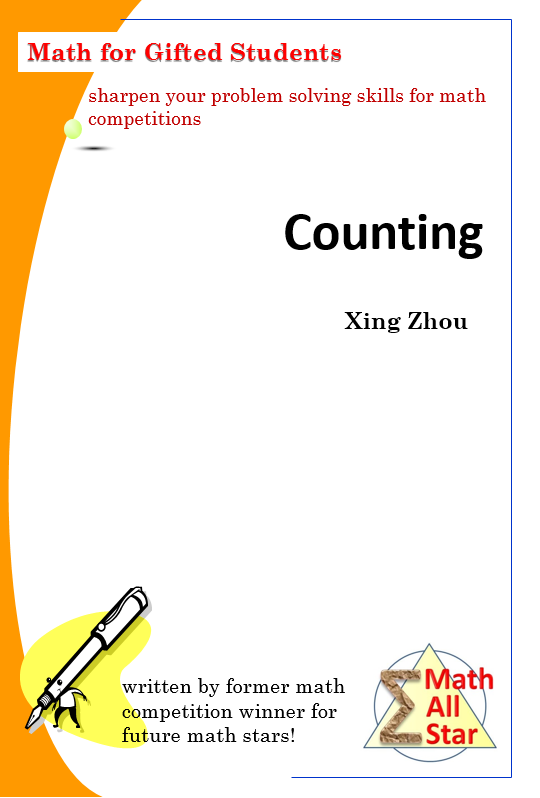Counting (Combinatorics) Ideal for Beginner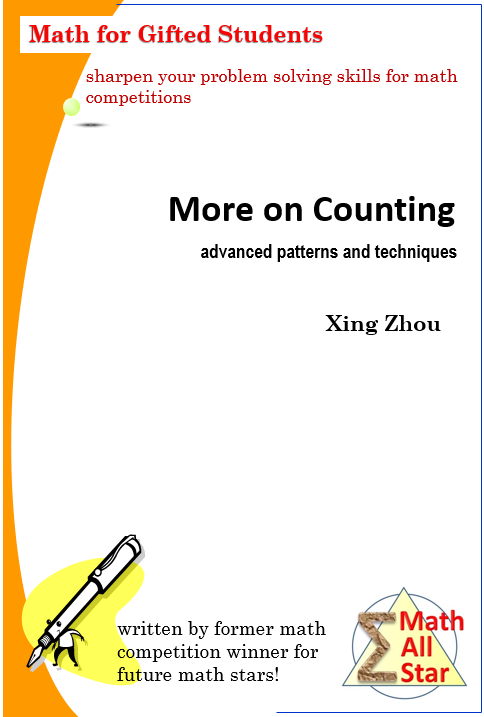More on Counting NEW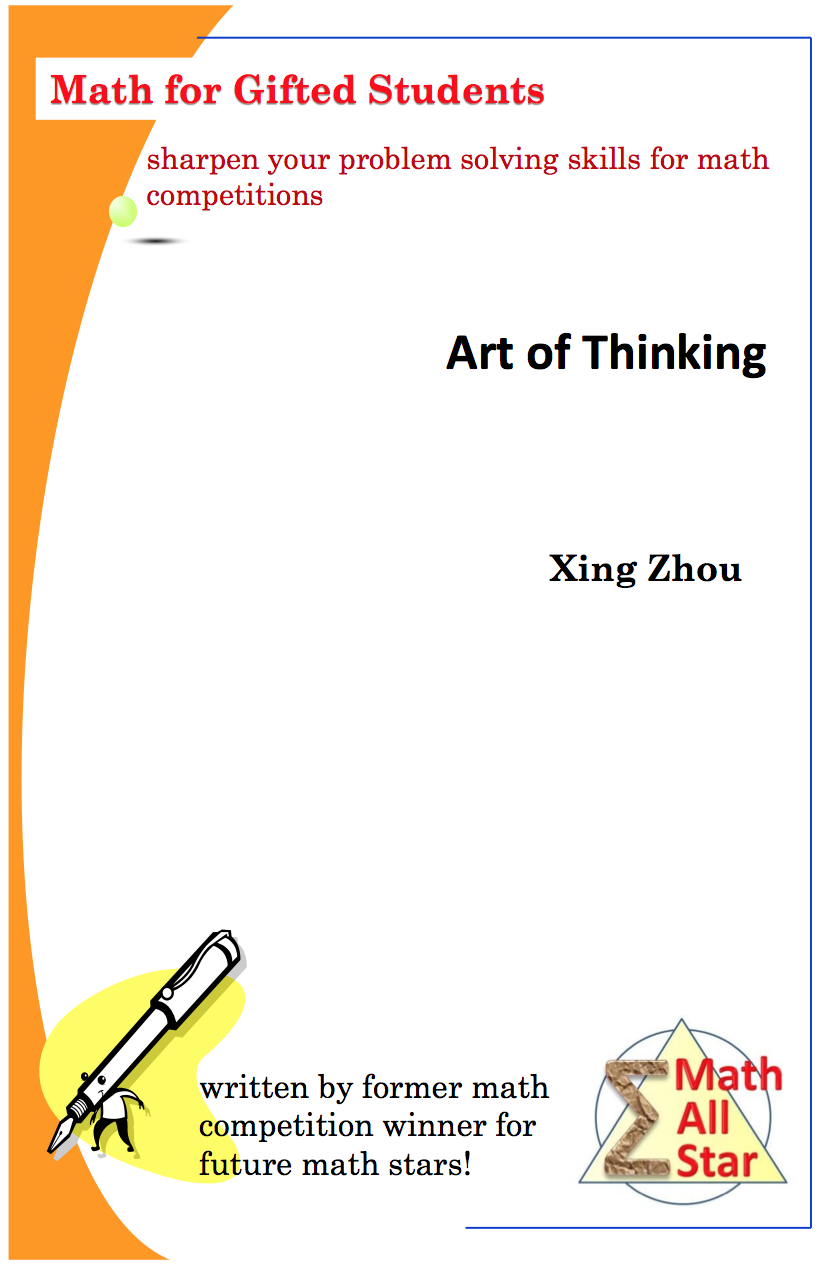Art of Thinking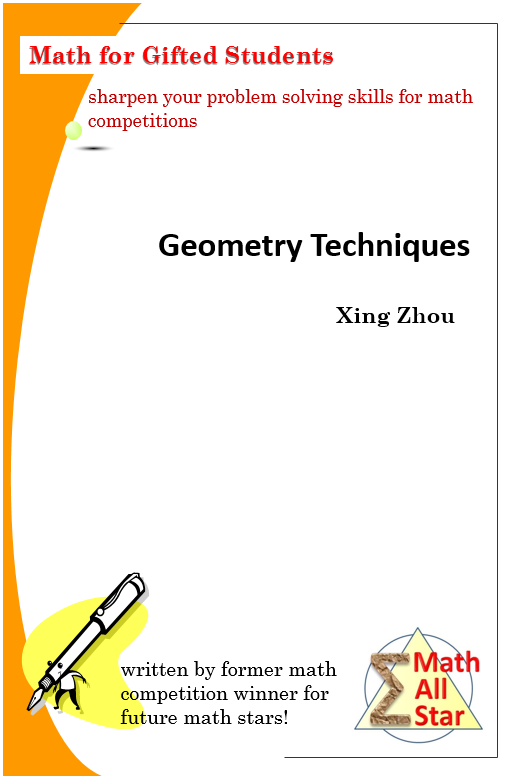Geometry TechniquesGeometry Theorems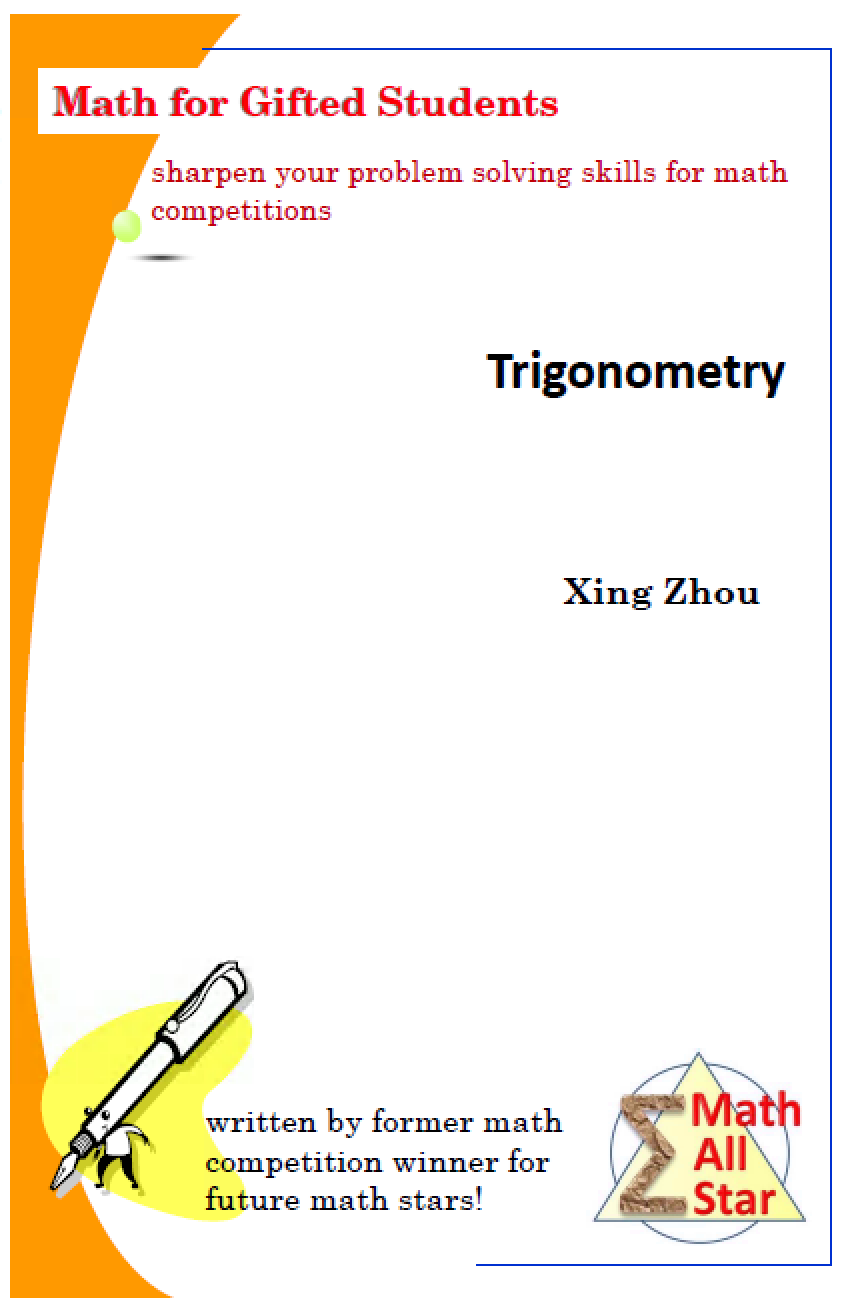Trigonometry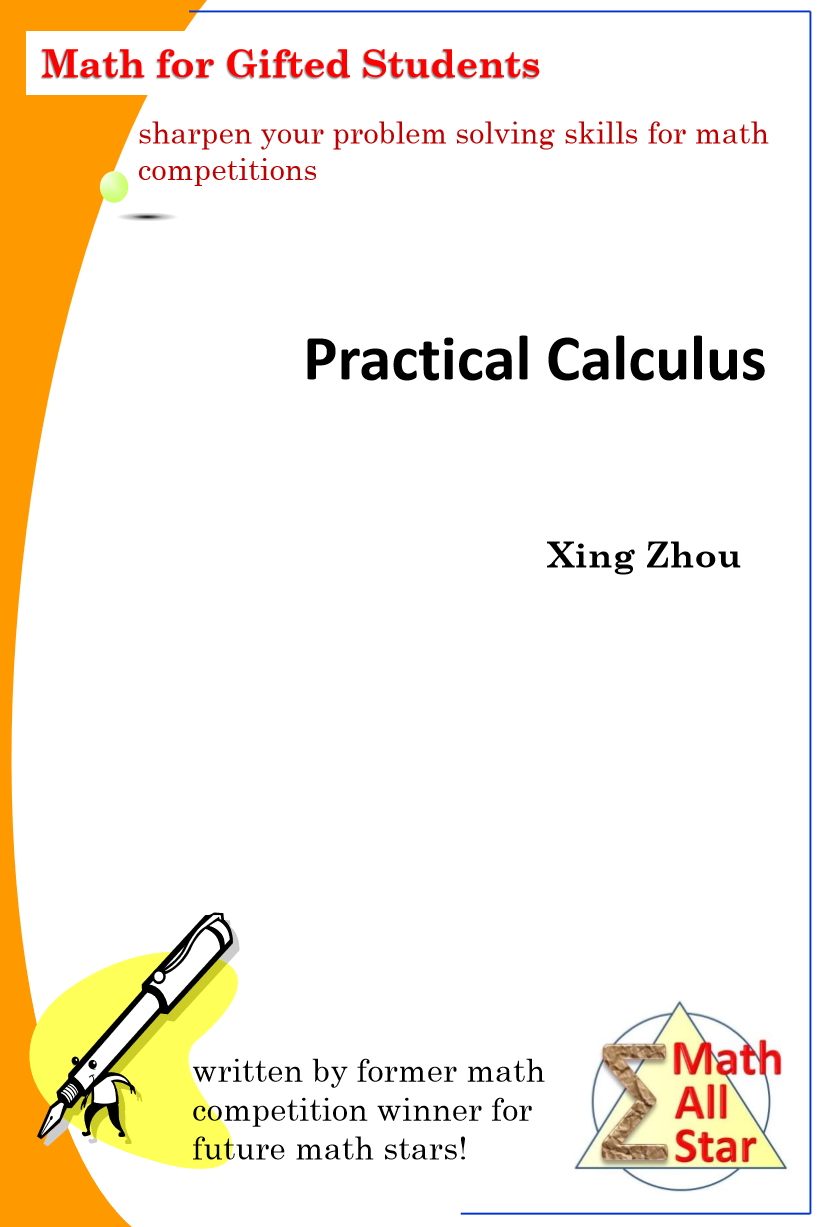Practical Calculus NEW

Practice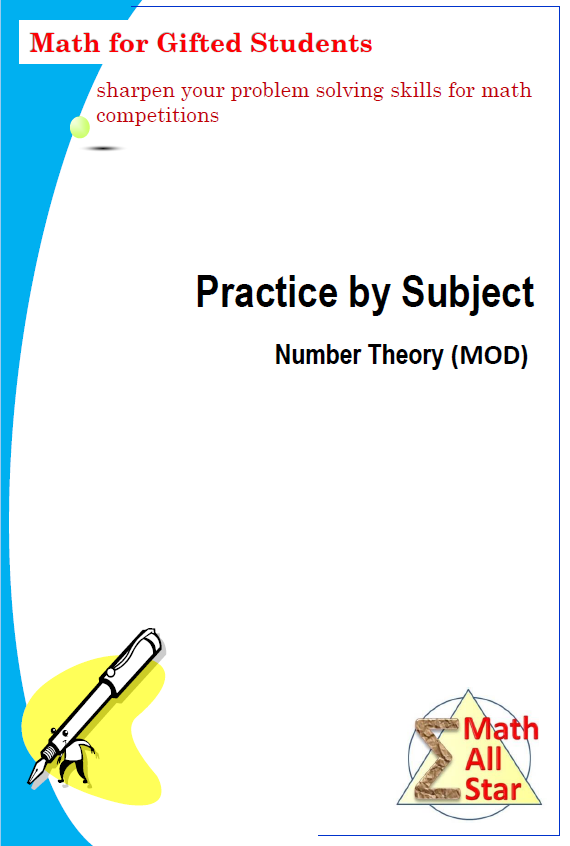Number Theory (MOD) Practice Popular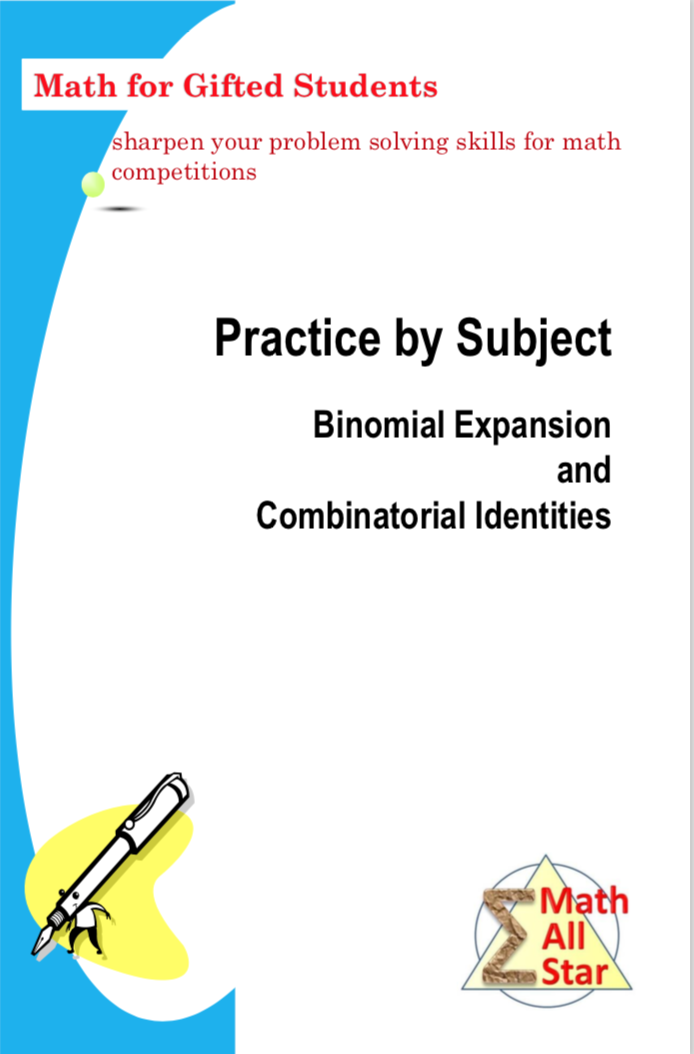Binomial Expansion and Combinatorial Identities Practice

Beyond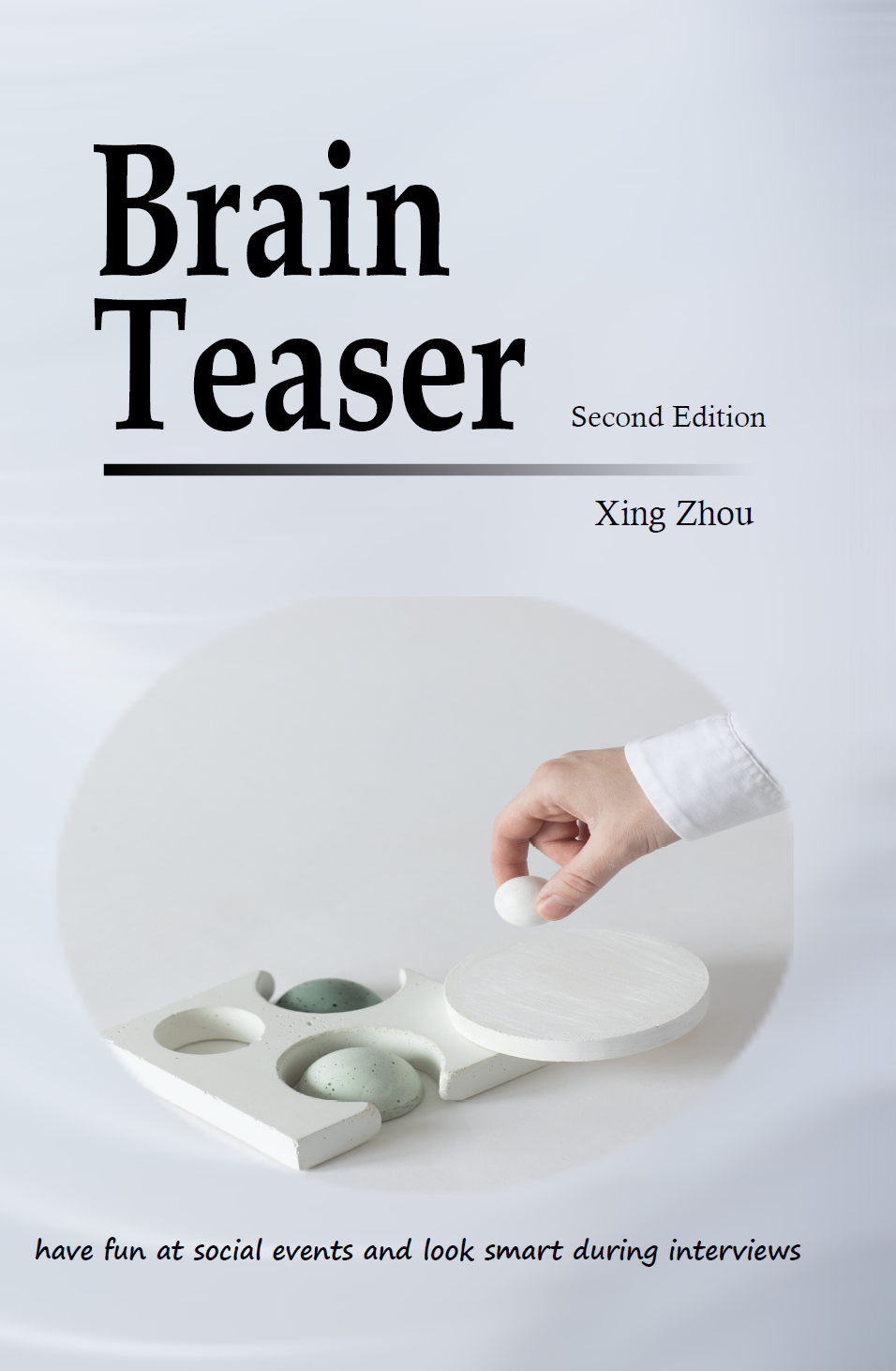Brain Teaser NEW